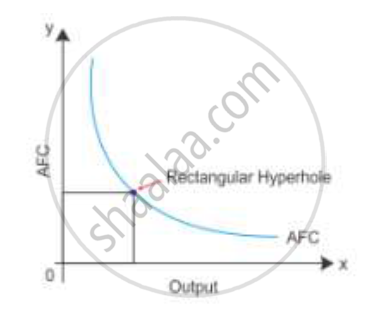# What is the Behaviour of Average Fixed Cost as Output is Increased? Why is It So? - Economics

What is the behaviour of average fixed cost as output is increased? Why is it so?

Explain with reason the behaviour of Average Fixed Cost as output is increased.

#### Solution

Average fixed cost is the fixed cost per unit of output. Average fixed cost curve slopes downward to the right. It shows that AFC decreases as output increases. It is a rectangular hyperbola curve. It means that the product of AFC and output is equal to TFC which remains constant at all levels of output.

TFC = AFC* Q

Therefore

AFC=(TFC)/QConcept: Cost - Average Fixed Cost
Is there an error in this question or solution?
2011-2012 (March) Delhi Set 1
Share